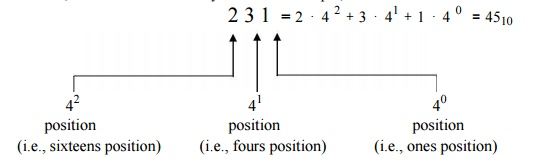Home | | Basic Electrical and Electronics Engineering | The Binary Number System

# The Binary Number System

When the base-10 child reached nine (the highest symbol in his scheme), the next penny gave him â€śone group of tenâ€ť, denoted as 10, where the â€ś1â€ť indicated one collection of ten.

The Binary Number System

Consider again the example of a child counting a pile of pennies, but this time in binary.

He would begin with the first penny: â€ś1.â€ť The next penny counted makes the total one single group of two pennies. What number is this?

When the base-10 child reached nine (the highest symbol in his scheme), the next penny gave him â€śone group of tenâ€ť, denoted as 10, where the â€ś1â€ť indicated one collection of ten.

Similarly, when the base-2 child reaches one (the highest symbol in his scheme), the next penny gives him â€śone group of twoâ€ť, denoted as 10, where the â€ś1â€ť indicates one collection of two.

Back to the base-2 child: The next penny makes one group of two pennies and one additional penny: â€ś11.â€ť The next penny added makes two groups of two, which is one group of 4: â€ś100.â€ť The â€ś1â€ť here indicates a collection of two groups of two, just as the â€ś1â€ť in the base-10 number 100 indicates ten groups of ten.

Upon completing the counting task, base -2 child might find that he has one group of four pennies, no groups of two pennies, and one penny left over: 101 pennies. The child counting the same pile of pennies in base-10 would conclude that there were 5 pennies. So, 5 in base-10 is equivalent to 101 in base-2. To avoid confusion when the base in use if not clear from the context, or when using multiple bases in a single expression, we append a subscript to the number to indicate the base, and write:

510 =1012

Just as with decimal notation, we write a binary number as a string of symbols, but now each symbol is a 0 or a 1. To interpret a binary number, we multiply each digit by the power of 2 associated with that digitâ€™s position.

For example, consider the binary number 1101. This number is:Since binary numbers can only contain the two symbols 0 and 1, numbers such as 25 and 1114000 cannot be binary numbers.

We say that all data in a computer is stored in binaryâ€”that is, as 1â€™s and 0â€™s. It is important to keep in mind that values of 0 and 1 are logical values, not the values of a physical quantity, such as a voltage. The actual physical binary values used to store data internally within a computer might be, for instance, 5 volts and 0 volts, or perhaps 3.3 volts and 0.3 volts or perhaps reflection and no reflection. The two values that are used to physically store data can differ within different portions of the same computer. All that really matters is that there are two different symbols, so we will always refer to them as 0 and 1.

A string of eight bits (such as 11000110) is termed a byte. A collection of four bits (such as 1011) is smaller than a byte, and is hence termed a nibble. (This is the sort of nerd-humor for which engineers are famous.)

The idea of describing numbers using a positional system, as we have illustrated for base-10 and base-2, can be extended to any base. For example, the base-4 number 231 is:Study Material, Lecturing Notes, Assignment, Reference, Wiki description explanation, brief detail
Basic Electrical and electronics : Digital Electronics : The Binary Number System |